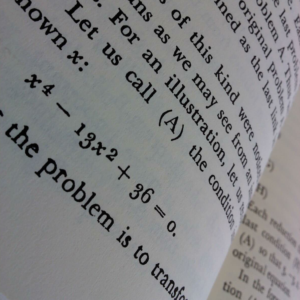## C.3 Lowercase Numbers in Mathematics

The ten digits, like the letters of the alphabet, have both uppercase and lowercase forms.

 Lowercase: $0123456789$ Uppercase: 0123456789

The lowercase digits are also known as ‘text figures’ or ‘old style figures’.

Lowercase numbers aren’t as common as they once used to be but you will find them in any well-typeset book for the same reason that WE DON’T WRITE IN ALL CAPS: uppercase looks out of place and shouty.

Some people say that although lowercase digits look better for page numbers, dates, and numbers here and there in text, they shouldn’t be used in mathematics or in tables of numbers. But really there is no good reason why they shouldn’t.

You can find handwriting samples of great mathematicians such as Euler, Pascal, Newton, and Leibniz – they all used lowercase numbers when writing mathematics. Tables of numbers were written in lowercase until recently. My copy of How to Solve It by Polya has lowercase numbers.Aside from the typographic argument that lowercase numbers are more aesthetically pleasing because they blend better with letters, they just look... cooler. I like old-fashioned things, so lowercase numbers are what I’m going to use.

In case other people want to know how to use lowercase digits in mathematics, I’d like to share my configurations of LaTeX, MetaPost, and MathJax that make this possible.

### C.3.1 LaTeX

For LaTeX, the following code in the preamble will make digits lowercase in most cases:

\usepackage{eco} %Lowercase digits outside of math mode in the computer modern font

%Use lowercase digits in math mode
\DeclareMathSymbol{0}\mathalpha{letters}{0}
\DeclareMathSymbol{1}\mathalpha{letters}{1}
\DeclareMathSymbol{2}\mathalpha{letters}{2}
\DeclareMathSymbol{3}\mathalpha{letters}{3}
\DeclareMathSymbol{4}\mathalpha{letters}{4}
\DeclareMathSymbol{5}\mathalpha{letters}{5}
\DeclareMathSymbol{6}\mathalpha{letters}{6}
\DeclareMathSymbol{7}\mathalpha{letters}{7}
\DeclareMathSymbol{8}\mathalpha{letters}{8}
\DeclareMathSymbol{9}\mathalpha{letters}{9}


When using the mathpazo package I found the following code necessary:

\usepackage[osf]{mathpazo} %Lowercase digits outside of math mode

%Use lowercase digits in math mode
\DeclareSymbolFont{osf}{OT1}{pplj}{m}{n}
\DeclareMathSymbol{0}\mathalpha{osf}{0}
\DeclareMathSymbol{1}\mathalpha{osf}{1}
\DeclareMathSymbol{2}\mathalpha{osf}{2}
\DeclareMathSymbol{3}\mathalpha{osf}{3}
\DeclareMathSymbol{4}\mathalpha{osf}{4}
\DeclareMathSymbol{5}\mathalpha{osf}{5}
\DeclareMathSymbol{6}\mathalpha{osf}{6}
\DeclareMathSymbol{7}\mathalpha{osf}{7}
\DeclareMathSymbol{8}\mathalpha{osf}{8}
\DeclareMathSymbol{9}\mathalpha{osf}{9}


### C.3.2 MetaPost

To keep MetaPost labels consistent with the way LaTeX is typeset, I keep all LaTeX commands that would alter the appearance of diagram labels in a separate package file which I call ‘symbols.sty’, then load it with a file ‘texpre.tex’ containing this:

\documentclass[12pt]{article}
\usepackage{../packages/symbols} %points to symbols.sty
\begin{document}

which is loaded with the following line in MetaPost:
input TEX;
TEXPRE("%&latex\input{texpre}");

If you want to typeset labels in MetaPost without using TeX, you can specify the font to use like so:
defaultfont:="ecorm1200"; %Lowercase numbers in computer modern font

Or in palatino:
defaultfont:="pplr9o";
defaultscale := 12pt/fontsize defaultfont; %match 12pt font


### C.3.3 MathJax

For MathJax (a javascript library that typesets TeX on webpages), I use the following configuration to make digits lowercase:

MathJax.Hub.Register.StartupHook("TeX Jax Ready",function () {
var TEX = MathJax.InputJax.TeX;
for (i=0; i<10; i++) {
TEX.Definitions.special[i.toString()] = "myDigit";
};
TEX.Definitions.special["."] = "myDigit";
TEX.Parse.Augment({
myDigit: function (c) {
this.Push(this.mmlToken(MML.mi(c).With({mathvariant:MML.VARIANT.OLDSTYLE})));
}
});
});